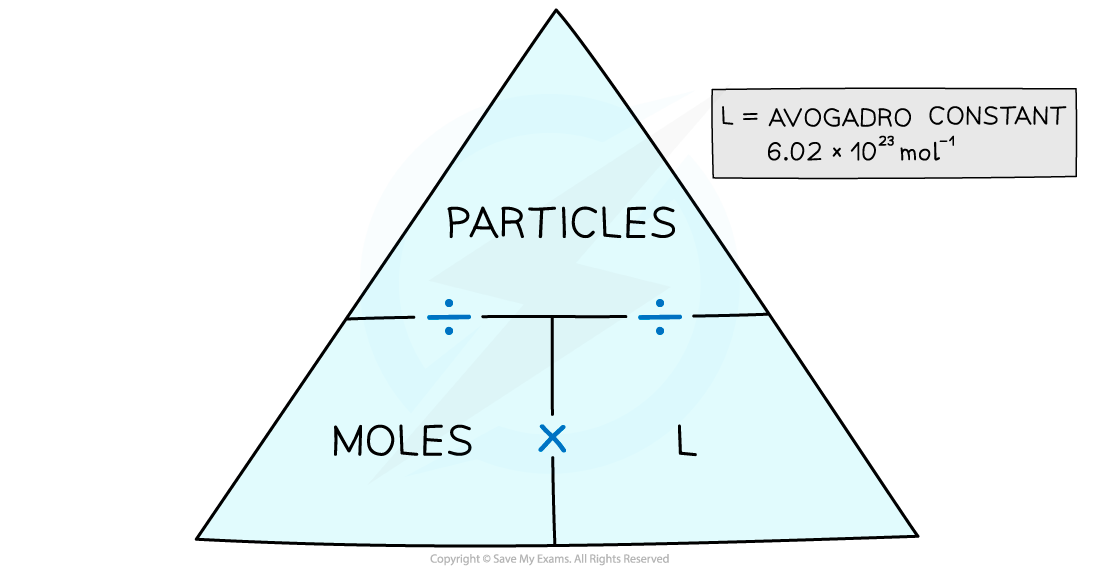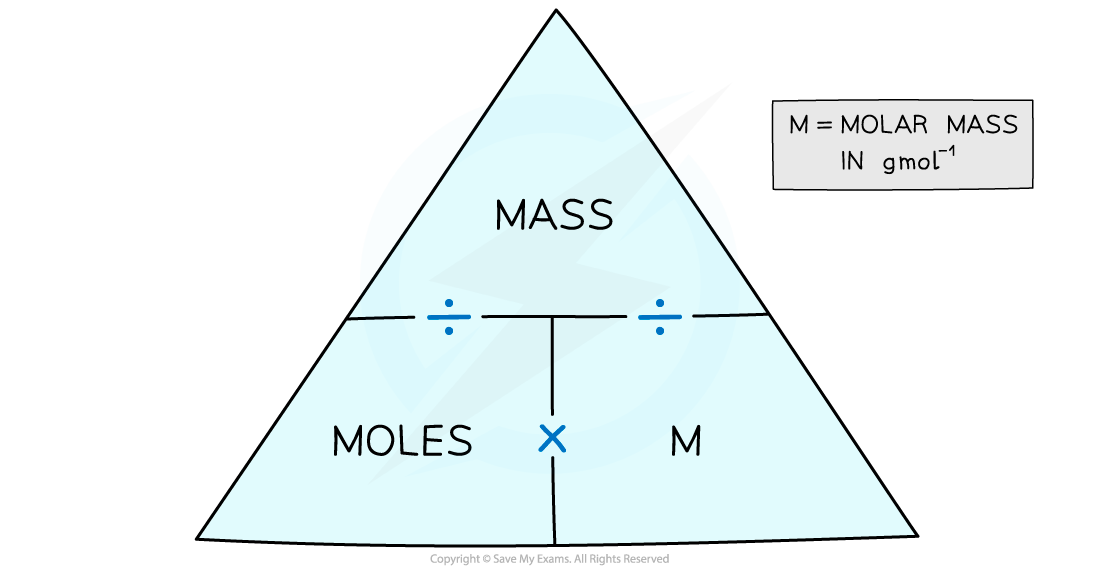# IB DP Chemistry: SL复习笔记1.1.5 Moles-Mass Problems

### Moles, Particles & Masses

• Since atoms are so small, any sensible laboratory quantity of substance must contain a huge number of atoms
• Such numbers are not convenient to work with, so using moles is a better unit to deal with the sort of quantities of substance normally being measured
• When we need to know the number of particles of a substance, we usually count the number of moles
• The number of moles or particles can be calculated easily using a formula triangleThe moles and particles formula triangle – cover with your finger the one you want to find out and follow the directions in the triangle

#### Worked Example

How many hydrogen atoms are in 0.010 moles of CH3CHO?

• There are 4 H atoms in 1 molecule of CH3CHO
• So, there are 0.040 moles of H atoms in 0.010 moles of CH3CHO
• The number of H atoms is the amount in moles x L
• This comes to 0.040 x (6.02 x 1023) = 2.4 x 1022 atoms

#### Worked Example

How many moles of hydrogen atoms are in 3.612 x 1023 molecules of H2O2?

• In 3.612 x 1023 molecules of H2O2  there are 2 x (3.612 x 1023) atoms of H
• So, there are 7.224 x 1023 atoms of H
• The number of moles of H atoms is the number of particles ÷ L
• This comes to 7.224 x 1023 ÷ (6.02 x 1023) = 1.20 moles of H atoms

#### Moles and Mass

• We count in moles by weighing the mass of substances
• The number of moles can be calculated by using a formula triangleThe moles and mass formula triangle – cover with your finger the one you want to find out and follow the directions in the triangle

#### Worked Example

What is the mass of 0.250 moles of zinc?

• From the periodic table the relative atomic mass of Zn is 65.38
• So, the molar mass is 65.38 g mol-1
• The mass is calculated by moles x molar mass
• This comes to 0.250 mol x 65.38 g mol-1 = 16.3 g

#### Worked Example

How many moles are in 2.64 g of sucrose, C12H11O22  (Mr = 342.3)?

• The molar mass of sucrose is 342.3 g mol-1
• The number of moles is found by mass ÷ molar mass
• This comes to  2.64 g ÷ 342.3 g mol-1 = 7.71 x 10-3 mol

#### Exam Tip

Always show your workings in calculations as its easier to check for errors and you may pick up credit if you get the final answer wrong.